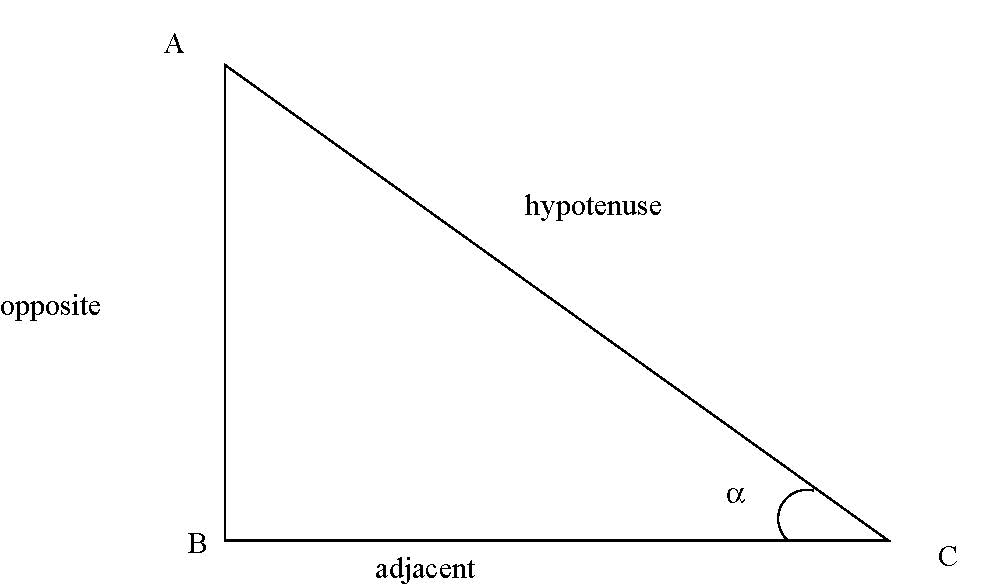$$\newcommand{\id}{\mathrm{id}}$$ $$\newcommand{\Span}{\mathrm{span}}$$ $$\newcommand{\kernel}{\mathrm{null}\,}$$ $$\newcommand{\range}{\mathrm{range}\,}$$ $$\newcommand{\RealPart}{\mathrm{Re}}$$ $$\newcommand{\ImaginaryPart}{\mathrm{Im}}$$ $$\newcommand{\Argument}{\mathrm{Arg}}$$ $$\newcommand{\norm}{\| #1 \|}$$ $$\newcommand{\inner}{\langle #1, #2 \rangle}$$ $$\newcommand{\Span}{\mathrm{span}}$$

# 0.3: Trigonometry

•• Contributed by Pamini Thangarajah
• Professor (Mathematics & Computing) at Mount Royal University
$$\newcommand{\vecs}{\overset { \rightharpoonup} {\mathbf{#1}} }$$ $$\newcommand{\vecd}{\overset{-\!-\!\rightharpoonup}{\vphantom{a}\smash {#1}}}$$$$\newcommand{\id}{\mathrm{id}}$$ $$\newcommand{\Span}{\mathrm{span}}$$ $$\newcommand{\kernel}{\mathrm{null}\,}$$ $$\newcommand{\range}{\mathrm{range}\,}$$ $$\newcommand{\RealPart}{\mathrm{Re}}$$ $$\newcommand{\ImaginaryPart}{\mathrm{Im}}$$ $$\newcommand{\Argument}{\mathrm{Arg}}$$ $$\newcommand{\norm}{\| #1 \|}$$ $$\newcommand{\inner}{\langle #1, #2 \rangle}$$ $$\newcommand{\Span}{\mathrm{span}}$$ $$\newcommand{\id}{\mathrm{id}}$$ $$\newcommand{\Span}{\mathrm{span}}$$ $$\newcommand{\kernel}{\mathrm{null}\,}$$ $$\newcommand{\range}{\mathrm{range}\,}$$ $$\newcommand{\RealPart}{\mathrm{Re}}$$ $$\newcommand{\ImaginaryPart}{\mathrm{Im}}$$ $$\newcommand{\Argument}{\mathrm{Arg}}$$ $$\newcommand{\norm}{\| #1 \|}$$ $$\newcommand{\inner}{\langle #1, #2 \rangle}$$ $$\newcommand{\Span}{\mathrm{span}}$$

## The right triangle

Trigonometric functionsUsually in a triangle when there is no chance of ambiguity, the sides opposite to each vertex have length denoted by the corresponding small letter.In the diagram, the triangle $$ABC$$ has a right angle at $$B.$$

$$\sin (A)= \frac{\mbox {length of the opposite side}}{ \mbox{ length of the hypotenuse}}=\frac{a}{b}.$$
$$\cos (A)= \frac{\mbox {length of the adjacent side}}{ \mbox{ length of the hypotenuse}}=\frac{c}{b}.$$
$$\tan (A)= \frac{\mbox {length of the opposite side}}{ \mbox{ length of the length of the adjacent side}} =\frac{a}{c}= \frac{\sin (A)}{\cos (A)}.$$
The remanining functions can be considered to be reciprocal functions.
$$\csc (A)=\frac{1}{\sin (A)},$$
$$\sec (A)=\frac{1}{\cos (A)},$$
$$\cot (A)=\frac{1}{\tan (A)}.$$

## Solving the general triangle

Rules

Consider the triangle $$ABC$$ in the diagram:1. The sine law:
$$\frac{a}{ \sin (A)}=\frac{b}{ \sin (B)}=\frac{c}{ \sin (C)}$$
2. The cosine law:
$$a^2=b^2+c^2-2bc \cos (A)$$ or equivalently,
$$\cos (A)= \frac{b^2+c^2-a^2}{2bc}$$
3. The area of the triangle $$ABC$$ is $$\frac{1}{2} bc \sin (A).$$
4. If the perimeter of the triangle $$= 2s= a+b+c,$$ then $$\sin (A)= \frac{2}{bc} \sqrt{s(s-a)(s-b)(s-c)}$$
and the area of the triangle is $$\sqrt{s(s-a)(s-b)(s-c)}$$.

## Unit Circle

Definition

Unit circle is circle with center at the origin $$(0,0)$$ and radius $$1$$.
Unit circle has the equation $$x^2+y^2=1.$$The radian measure of an angle is equal to the numerical value of the length of the arc of the unit circle from the point
$$(1,0)$$ to the point $$(x,y).$$Thus, $$\mbox{length of the arc } AP=\alpha \mbox{ radian units}.$$
Hence we obtain the conversion from degrees to radians and vice-versa:$$\pi \,{\rm radians}=180^{\circ}$$

### Definition of the trigonometric functions

There are two ways to define trigonometric functions:

Definition

Using triangles or circles.

1. The definition using triangles is too restrictive in that it treats only angles between $$0$$ degree and $$90$$ degrees.

2. The definition using circles, on the other hand, leads to a much more general definition, since the angle can now take on any real value whatsoever.In the diagram above, $$A(1,0)$$ is the fixed point from which measurements begin. Angles and arc lengths are measured from $$A$$ in a counter-clockwise direction.

If $$P(x,y)$$ is any point on the circumference of the unit circle, then if the arc length of $$AP= \alpha$$ units, then $$\angle AOP = \alpha$$ radians, we define $$\sin (\alpha)=x \mbox{ and} \cos (\alpha)=y.$$

Further, we define $$\tan (\alpha)=\frac{\sin (\alpha)}{\cos (\alpha)}$$
$$\cot (\alpha)=\frac{\cos (\alpha)}{\sin (\alpha)},$$
$$\sec (\alpha)=\frac{1}{\cos (\alpha)},$$
$$\csc (\alpha)=\frac{1}{\sin (\alpha)}.$$

### Some important relationships on unit circle

Rules

$$\left.\begin{array}{c} \sin (\pi- \alpha)=\sin (\alpha).\\ \cos (\pi- \alpha)=-\cos (\alpha).\\ \tan (\pi- \alpha)=-\tan (\alpha). \end{array} \right\} \mbox{ Quadrant II}$$

$$\left.\begin{array}{c} \sin (\pi+\alpha)=-\sin (\alpha).\\ \cos (\pi+ \alpha)=-\cos (\alpha).\\ \tan (\pi+ \alpha)=\tan (\alpha). \end{array} \right\} \mbox{ Quadrant III}$$

$$\left.\begin{array}{c} \sin (2\pi- \alpha))=\sin (-\alpha)=-\sin (\alpha).\\ \cos (2\pi- \alpha)=\cos (-\alpha=\cos (\alpha).\\ \tan (2\pi- \alpha)=\tan (-\alpha)=-\tan (\alpha). \end{array} \right\} \mbox{ Quadrant IV}$$

$$\sin (\pi/2-\alpha)=\cos (\alpha).$$
$$\cos (\pi/2-\alpha)=\sin (\alpha).$$

### Basic identities

Basic identities

\begin{eqnarray} \sin^2(A)+\cos^2(A)& =& 1.\\
1+\tan^2(A)&=&\sec^2(A).\\
1+\cot^2(A)&=&\csc^2(A).\\
\cos(A+B)&=&\cos(A)\cos(B)-\sin(A) \sin(B).\\
\cos(A-B)&=&\cos(A)\cos(B)+\sin(A) \sin(B).\\
\sin(A+B)&=&\sin(A)\cos(B)+\cos(A) \sin(B).\\
\sin(A-B)&=&\sin(A)\cos(B)-\cos(A) \sin(B).\\
\sin(2A)&=&2 \sin(A) \cos (A).\\
\cos(2A)&=& \cos^2(A)- \sin^2(A)\\
&=& 2 \cos^2(A)-1\\
&=& 1-2 \sin^2(A).\\
\tan(A+B)&= &\displaystyle\frac{\tan (A) + \tan (B)}{1-\tan (A) \tan (B)}.\\
\tan(A-B)&= &\displaystyle\frac{\tan (A) - \tan (B)}{1+\tan (A) \tan (B)}.\\
\tan(2A)&= &\displaystyle\frac{2\tan (A) }{1-\tan^2 (A) }.\\
\end{eqnarray}